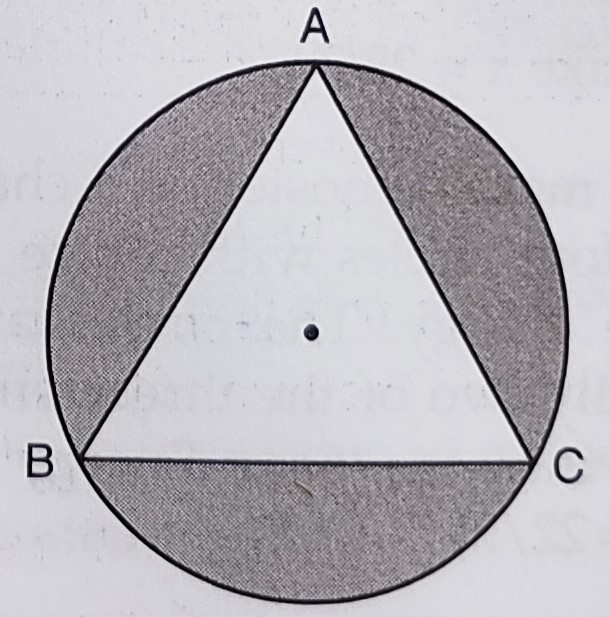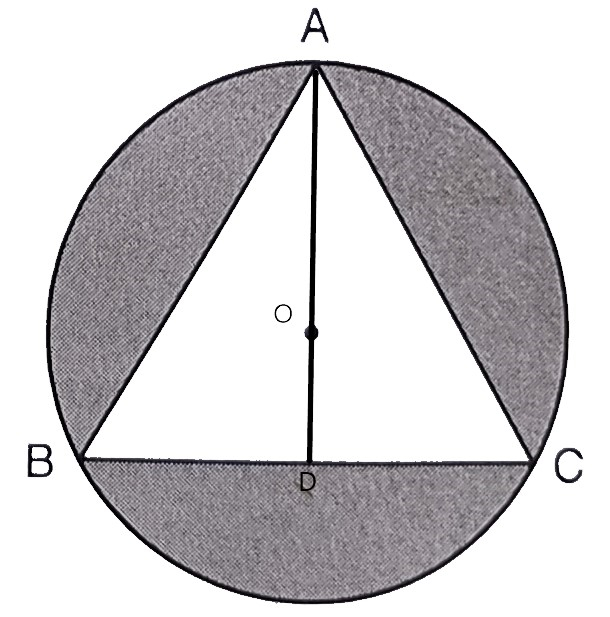"
">

# In the below figure, an equilateral triangle $A B C$ of side $6 \mathrm{~cm}$ has been inscribed in a circle. Find the area of the shaded region. (Take $\pi=3.14)$"

Given:

An equilateral triangle $A B C$ of side $6 \mathrm{~cm}$ has been inscribed in a circle.

To do:

We have to find the area of the shaded region.

Solution:

Length of the side of equilateral triangle $\mathrm{ABC}=6 \mathrm{~cm}$

Area of the equilateral triangle $=\frac{\sqrt{3}}{4} \times(6)^{2}$

$=\frac{\sqrt{3}}{4} \times 36$

$=9 \sqrt{3} \mathrm{~cm}^{2}$

$=9 \times 1.732$

$=15.588 \mathrm{~cm}^{2}$Draw $AD \perp BC$ which passes through the centre of the circle at $O$.

This implies,

$\mathrm{OA}=\frac{2}{3} \mathrm{AD}$               ($O$ is the centroid)

$=\frac{2}{3} \times \frac{\sqrt{3}}{2} \times 6$

$=2 \sqrt{3}\ cm$

Radius of the circle $r=2 \sqrt{3} \mathrm{~cm}$

Therefore,

Area of the circle $=\pi r^{2}$

$=\frac{22}{7} \times(2 \sqrt{3})^{2}$

$=\frac{22}{7} \times 12$

$=\frac{264}{7}$

$=37.714 \mathrm{~cm}^{2}$

Area of the shaded portion $=37.714-15.588$

$=22.126 \mathrm{~cm}^{2}$

The area of the shaded region is $22.126\ cm^2$.

Updated on: 10-Oct-2022

84 Views